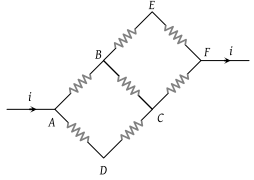In the adjoining circuit diagram each resistance is of 10 Ω. The current in the arm AD will be(1) $\frac{2i}{5}$

(2) $\frac{3i}{5}$

(3) $\frac{4i}{5}$

(4) $\frac{i}{5}$